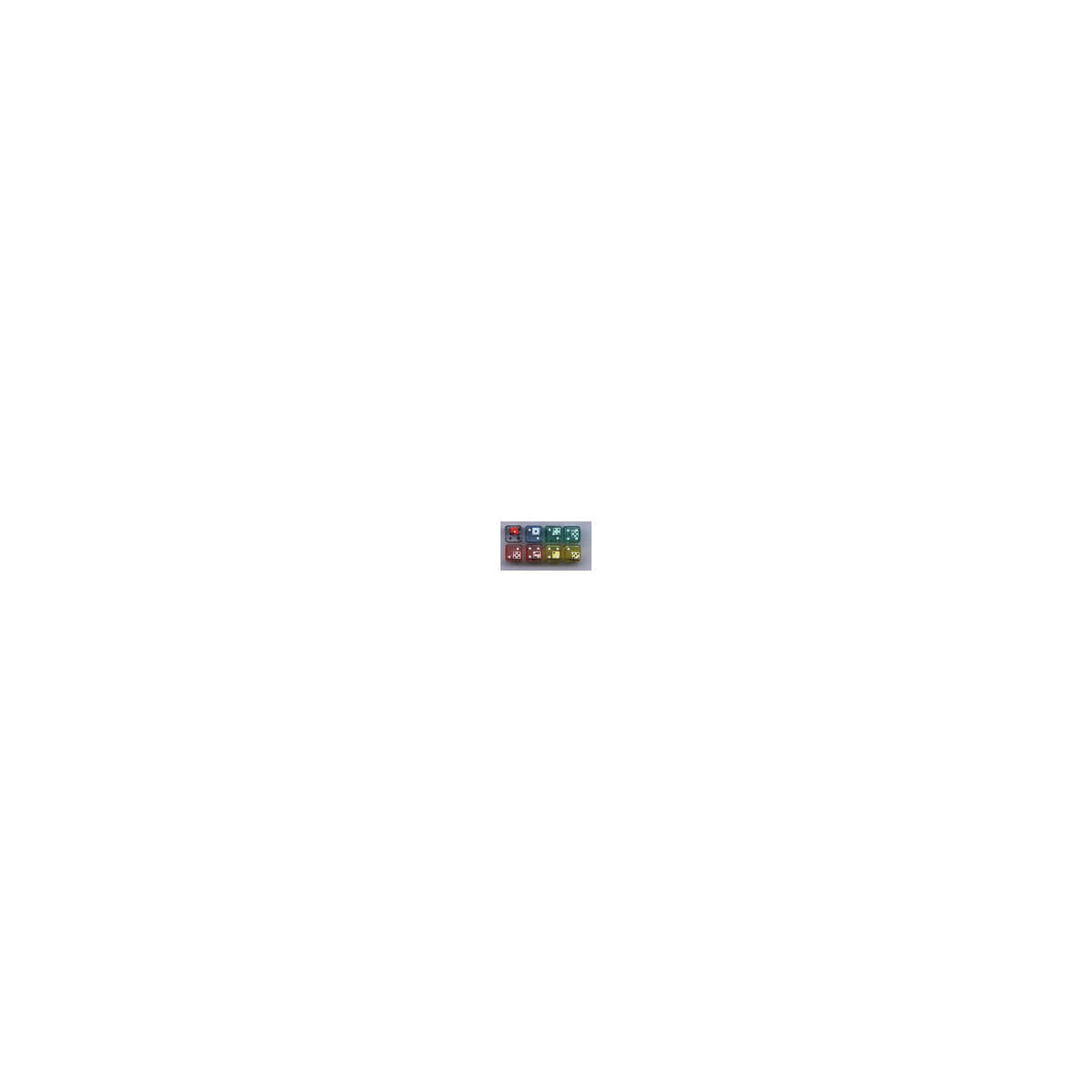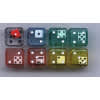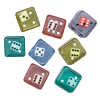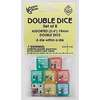•••# 6-Sided Double Dice, Set of 8

Product Number: KOP11703
A die inside of a die–great for math games! Roll it and add, subtract, multiply or divide the numbers. Assorted colors. Set o...
Quantity

A die inside of a die–great for math games! Roll it and add, subtract, multiply or divide the numbers. Assorted colors. Set of 8.
KOP11703